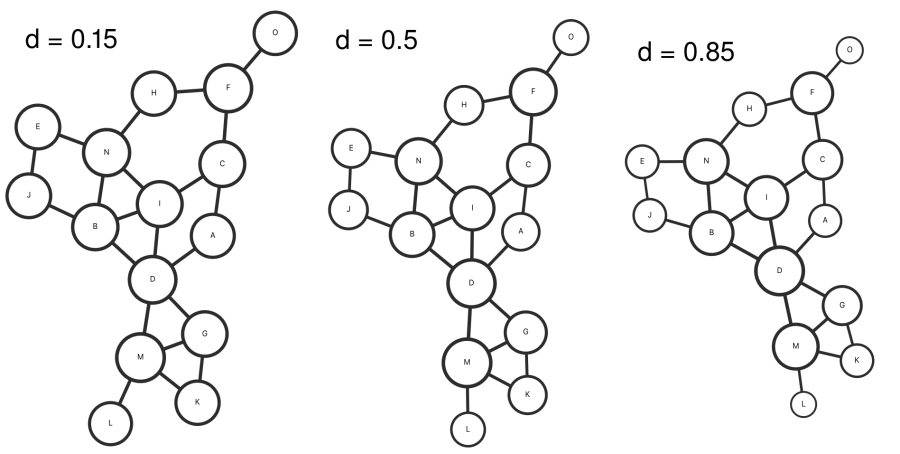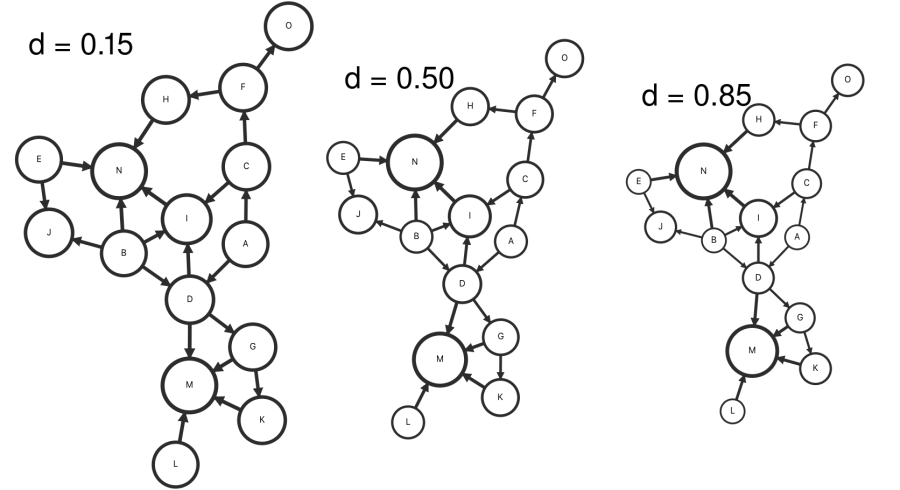# PageRank: Bringing Order To The Web

Investigating the OG of iterative centrality metrics

2020-02-27

Just a couple of decades ago, when the web was exploding with content, PageRank became its central nervous system, routing queries to high-quality and relevant pages through Google's search engine. Here I take a visual approach to evaluating the algorithm.

## In The Beginning ...

Admittedly, I struggle to recall the difficulty of finding relevant content on the internet during the late 1990s. Reading over the paper "The Anatomy of a Large-Scale Hypertextual Web Search Engine" gives a stark reminder of just how dire the situation was. Published in 1998 by Sergey Brin and Larry Page while founding a small company called Google, the paper discusses the inadequacy of search engines at the time. The primary engines, such as Yahoo, were using human maintained indices to catalog relevant content. Often, queries would return many pages of slightly relevant but entirely unsatisfactory web sites triggered by a simple keyword match. Even with a large, up-to-date index, it is easy to see that the sheer volume of content would rapidly outpace the ability of anyone to search the web using an index alone.

Anyone who has used a search engine recently (1998), can readily testify that the completeness of the index is not the only factor in the quality of search results.

The missing piece was the search engine's ability to rank content so that it could prioritize high-quality results in addition to query relevancy. To meet this challenge, the authors begin their investigation by viewing the web as a large directed graph where the nodes are web pages, and the edges are formed by hyperlinks from one page to another. By organizing online content into a graph, an analysis can be implemented to rank the content in terms of its importance. At the time, even academic efforts were limited to simple centrality metrics such as counting the number of linked pages, or the in-degree. While offering an improvement over the index alone, the in-degree metric gives weak results and is prone to manipulation.

Academic citation literature has been applied to the web, largely by counting citations or backlinks to a given page.

After establishing the poor state of search engines, the authors introduce their novel PageRank algorithm. In the ambitiously titled Section 2.1, "PageRank: Bringing Order to the Web," the golden ticket of the soon-to-be billion-dollar company is exhibited in glorious detail. On a slightly related note, I am astonished and impressed how aptly the name PageRank fits the intended purpose of the algorithm while also immortalizing the namesake of its progenitor!

## A Random Walker

PageRank is devised such that the rank of a node is influenced by the rank of neighboring nodes. Additionally, the ranks of the nodes in a graph should total to unity. Expressed as a formula, the PageRank ($PR$) of a node ($A$) is given by the sum of two terms. The first term is a constant, and the second is a linear combination of the ranks of linked nodes ($T$) normalized by the number of links directed out of node $T$. My custom notation $T\rightarrow A$ represents the set of nodes with edges pointing to $A$. Given this implicit formulation, the PageRank is solved iteratively by updating the rank of each node until the balance is satisfied within a specified precision at every node. The constant $d$ is a damping parameter that controls the "flow" of rank from one node into another. Counterintuitively, a larger damping parameter increases the rank transfer between nodes.

$PR(A) = \frac{(1-d)}{N} + d \sum_{T\rightarrow A} \frac{PR(T)}{C(T)}$

Conceptually, the PageRank has a nice interpretation as a probability distribution over the nodes of a graph, where the rank of a node gives the probability that a random walker would land on that node. As a hypothetical random walker traverses the graph, there are two types of moves it can make. Either it makes a jump to a completely random node within the graph, or it follows an edge from its current node to the next. Looking back at the formula, the first term $(1-d)/N$ gives the probability of the random jump, while the second term accounts for a walk across an edge. Intuitively, a more highly-connected node will have a higher probability of seeing the walker, and this effect is modulated by the damping parameter. While PageRank is not traditionally solved using a random walk, it provides an interesting interpretation. Additionally, modern graph-based recommendation engines often use random walks to calculate personalized rankings.

The normalizing factor in the summation $1/C(T)$ is given by the count of edges pointing out of the node ($T$). Interestingly, without this factor, the algorithm would be open to abuse by websites that have a million hyperlinks pointing to pages that they would like to promote. By dividing by this count, the contribution of each link is diminished by the addition of more links. As a side note, there is a typo in the original publication where the dividing factor $1/N$ was left out of the formulation. This normalization is certainly required to maintain a probability distribution.

## The Damping Parameter

It's interesting what type of role the damping parameter plays in the PageRank results. For pedagogical purposes, I choose a simple graph that can be easily visualized in just two dimensions. The graph is rendered using my own code that leverages the D3 library. The area of each node scales linearly with its PageRank to help compare differences when scanning the damping parameter.

In the first scan, the graph is taken to be undirected so that rank flows in both directions across edges during iteration. At a small value ($d=0.15$), the nodes are mostly uniform, as the first term $(1-d)/N$ dominates the balance. At a larger value ($d=0.85$), we can see from the size of the more well-connected nodes that they have an increased PageRank. Overall though, the damping parameter does not seem to have a substantial effect on the results in this case.Now looking at a directed graph, the effect of the damping parameter becomes more pronounced. The directional nature of the edges aligns more closely with the directional nature of hyperlinks on the web. Again, at a low damping parameter, the nodes are largely uniform, as expected. As the damping parameter is ramped up to large values, it's easier to see how the rank of a node is given by a combination of the rank of neighboring nodes. Almost as if the edges represent a "vote" whose weight scales with the rank of the contributing node.## To Conclude

While this analysis nicely illustrates the conceptual aspects of PageRank and the effect of its damping parameter, most practical applications use a much larger and interconnected graph that would require more complex visualization techniques. In these cases, the damping parameter likely has an even stronger effect than seen here. Even though modern search engines have evolved to a level of complexity that far surpasses the simple algorithm published in 1998, the ideas and techniques associated with the PageRank algorithm still have a place in many applications today, including personalized recommendation engines.

Sergey Brin and Lawrence Page, The Anatomy of a Large-Scale Hypertextual Web Search Engine, 1998